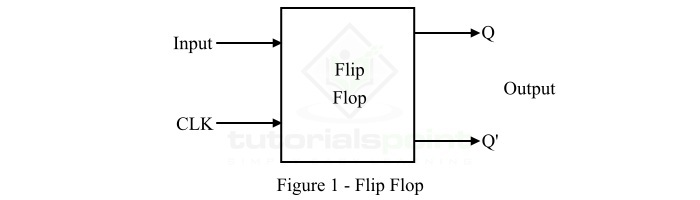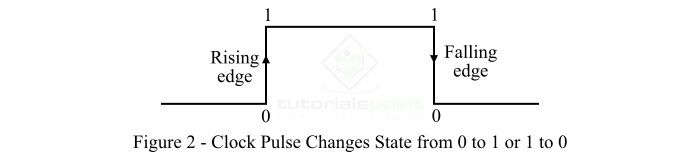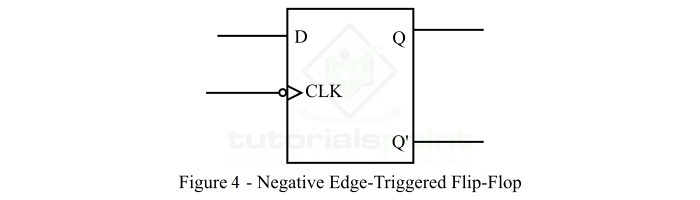# Edge-Triggered Flip-Flop

Let's start this article with a brief overview of basic flip-flops before moving onto discuss a special type of flip-flop called edge-triggered flip-flop, how it works, its types and applications.

## What is a Flip Flop?

In digital electronics, a flip-flop (FF) is a sequential logic circuit which is used for storing 1-bit of information. As we know, in digital systems, information is represented in binary form, i.e. in terms of 0 and 1. Where, a binary 0 and a binary 1 is referred to as a bit.

The flip flop is a 1-bit memory cell that stores information in terms of 0 or 1. Therefore, flip flop is the fundamental memory element in all digital systems.

A flip flop has one or more inputs and two outputs. Where, one is the normal output denoted by Q and the other is inverted output denoted by Q'. Hence, a flip flop has two stable states due to which it is also known as bistable multivibrator.

The logic symbol diagram of a typical flip-flop is shown in Figure-1.Based on the response to the clock pulse, flip flops are of two types namely, level-triggered flip flop and edge-triggered flip flop.

The type of flip flop whose operation is controlled by a continuous clock signal and its output state changes when the clock pulse is in a particular level either high or low is known as a level-triggered flip flop. On the other hand, the type of flip flop which respond when the clock change its state, i.e. either goes from high to low or low to high is known as an edgetriggered flip flop. We will discuss the edge-triggered flip flop in more detail in this article.

## What is an Edge-Triggered Flip-Flop?

The type of digital circuit which is capable of storing 1-bit of information and responds only when a specific edge of the clock pulse occurs is known as an edge-triggered flip-flop.

Therefore, the output state of the edge-triggered flip flop updates only when a specific edge of the clock pulse occurs, i.e. the clock pulse goes from either low to high or high to low states. This flip flop does not respond to a continuous clock pulse.

Edge-triggered flip-flop are used in several digital circuits where the output of the flip flop should be updated when the clock pulse changes its state from 0 to 1 or 1 to 0 as shown in Figure-2.Hence, the edge triggered flip flop operates only on the rising or falling edge of the clock pulse.

## Types of Edge-Triggered Flip-Flop

Depending on the responding edge of the clock pulse, the edge-triggered flip flops are classified into two types namely,

• Positive Edge-Triggered Flip-Flop

• Negative Edge-Triggered Flip-Flop

### Positive Edge-Triggered Flip-Flop

The type of edge-triggered flip-flop whose output changes its state only on the rising edge (edge that goes from low to high) of the clock pulse is called a positive edge-triggered flip-flop. The positive edge triggered flip flop is also called a rising edge-triggered flip-flop. The block diagram of a positive edge triggered flip flop is shown in Figure-3 below.In a positive edge triggered flip flop, the inputs are accepted and stored only when the clock pulse goes from low (0) to high (1), i.e. on the rising edge of the clock pulse. This stored value is then available on the outputs.

### Negative Edge-Triggered Flip-Flop

The type of edge-triggered flip flop whose output changes its state only on the falling edge (edge that goes from high to low) of the clock pulse is called a negative edge-triggered flip-flop. The negative edge triggered flip flop is also known as a falling edge-triggered flip-flop. The block diagram of a negative edge triggered flip flop is shown in Figure-4 below.In the case of negative edge triggered flip flop, the flip-flop captures and stores the inputs only when the clock pulse goes from high to low, i.e. on falling edge of the clock pulse.

## Operation of Edge-Triggered Flip-Flop

The operation of a typical edge-triggered flip-flop is described below −

In the edge-triggered flip-flop, the inputs are applied through the input terminals and a clock pulse is connected to the clock input of the flip-flop. The edge triggered flip flop responds according to the applied inputs when the clock pulse goes from either low to high or high to low. When this state transition of clock pulse occurs, the flip-flop captures and stores the input values. These stored input values will be then available on the outputs (Q and Q') of the flip-flop.

The important advantages of the edge-triggered flip flop are listed as follows −

• Edge triggered flip flops have an improved timing behavior as compared to the level triggered flip flops. This is because, the edge triggered flip flop responds only on the transition of clock pulse.

• Edge-triggered flip flops reduces the possibility of glitches that cause errors in the system.

• Edge triggered flip flops consumes relative low power than the level-triggered flip flops.

• Edge-triggered flip flop has relatively less complex circuit design.

• Edge triggered flip flops can be easily integrated in the form of digital ICs.

• Edge triggered flip flops are very useful in digital systems having very high clock speed.

## Applications of Edge-Triggered Flip-Flop

Edge triggered flip flops are used in a variety of digital systems. Some common applications of edge triggered flip flops are −

• Edge triggered flip-flops are used in registers to store and transfer binary information among different parts of a digital circuit.

• Edge triggered flip flops are used in digital counters for generating sequences of binary values.

• Edge triggered flip flops are also used for digital signal processing.

• Edge triggered flip flops are used in several digital applications where timing and signal synchronization is required.

• Edge-triggered flip flops are also used to build memory cells of ROM, RAM, etc. to stores binary data in a digital system.

## Conclusion

This is all about edge triggered flip-flop. In conclusion, edge triggered flip-flops are one of the important building blocks of many digital systems such as memories, registers, counters, etc.

Updated on: 24-Apr-2023

4K+ Views